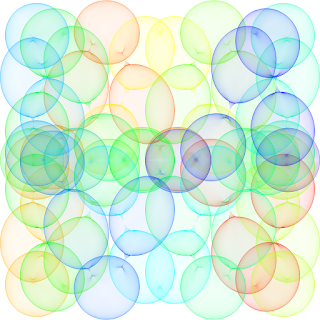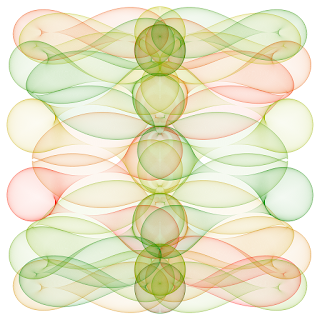Sunday, 20 September 2015

Math Art Work with just segments and circles

This post was inspired by the article in the CNN in the following link.

Using a big number of segments or circles we can create nice art work like the images below. When a number of segments under a certain parametrization intersect at some points, the set of intersection points define a curve on the plane called the evolute.

For example, imagine a fixed length bar which stands vertical on the Y-axis. Then, we take the extreme of the bar at (0,0) and move it through the X-axis, while letting the other extreme descend through the Y-axis. The intersection of all this segment will define a curve, and the segments are tangent to the curve at each of the intersection points. Many curves such as parabola, hyperbola can be constructed in this way.python mathart.py -c black -C black -n 100 -o "artwork10.png" -e line -X1 "0.0" -Y1 "sin(pi/2.0*k/n)*(-1.0)" -X2 "cos(pi/2.0*k/n)" -Y2 "0.0"

Using more complex parametrizations as the one described in the origina article and in the script.sh file, and letting our imagination fly, we can come up with nice geometrical figures.

When I first tackled this problem with the very first lines of code at the early stage of the program, I observed that the images I obtained where fuzzy. If I increased the number of elements to get more defined curves, what I got was large areas of pixels of the same color.  This is due to the fact that computers image are a set of discrete points instead of a continuum of points. When drawing many elements the non-integer coordenates will be plotted at the nearest pixel, creating an overlapping, as we can appreciate on the picture below. This effect is known in signal processing as the Aliasing problem.Example of figure without using anti-aliasing technique.

The solution to this problem is obviously to increase the resolution of the image.  In order to increase the resolution while preserving the same image size, what we do is to create a first image larger than our final image. All calculations are done in this larger image, larger by a factor specified on the command line of the program as super-sampling. Once we have this larger image, we resize or down-size the image to the size we originally wanted. In order to down-size the image, we can choose amongst several algorithms to apply: Nearest, Bilinear, Bicubic, Antialias. In this case we use the Antialias filtering which is the one resulting in higher quality images in this case.

Using this super-sampling technique, we come up with much better quality pictures. The pictures below have been created with the Python script at the end of this post. The legend of each pictures include the command-line used to create the picture. All these examples are under the script.sh file on the same directory.

You can get the code from my github repository at the following link.python mathart.py -c red -C blue -n 25000 -o "artwork1.png" -e line -X1 "sin(108.0*pi*k/n)*sin(4.0*pi*k/n)" -Y1 "cos(106.0*pi*k/n)*sin(4.0*pi*k/n)" -X2 "sin(104.0*pi*k/n)*sin(4.0*pi*k/n)" -Y2 "cos(102.0*pi*k/n)*sin(4.0*pi*k/n)"python mathart.py -c black -C blue -n 25000 -o "artwork2.png" -e line -X1 "3.0/4.0*cos(86.0*pi*k/n)" -Y1 "sin(84.0*pi*k/n)**5" -X2 "sin(82.0*pi*k/n)**5" -Y2 "3.0/4.0*cos(80.0*pi*k/n)"python mathart.py -c red -C blue -n 25000 -o "artwork3.png" -e circle -Xc "sin(14.0*pi*k/n)" -Yc "cos(26.0*pi*k/n)**3" -R "1.0/4.0*cos(40.0*pi*k/n)**2"python mathart.py -c red -C blue -n 25000 -o "artwork4.png" -e circle -Xc "cos(6.0*pi*k/n)" -Yc "sin(20.0*pi*k/n)**3" -R "1.0/4.0*cos(42.0*pi*k/n)**2"python mathart.py -c red -C blue -n 25000 -o "artwork5.png" -e circle -Xc "cos(6.0*pi*k/n)" -Yc "sin(14.0*pi*k/n)**3" -R "1.0/4.0*cos(66.0*pi*k/n)**2"python mathart.py -c black -C blue -n 25000 -o "artwork6.png" -e circle -Xc "cos(14.0*pi*k/n)**3" -Yc "sin(24.0*pi*k/n)**3" -R "1.0/3.0*cos(44.0*pi*k/n)**4"python mathart.py -c red -C green -n 25000 -o "artwork7.png" -e circle -Xc "sin(22.0*pi*k/n)**3" -Yc "cos(6.0*pi*k/n)" -R "1.0/5.0*cos(58.0*pi*k/n)**2"python mathart.py -c yellow -C blue -n 25000 -o "artwork8.png" -e circle -Xc "cos(2.0*pi*k/n)" -Yc "sin(18.0*pi*k/n)**3" -R "1.0/4.0*cos(42.0*pi*k/n)**2"

The Python code is include below.